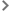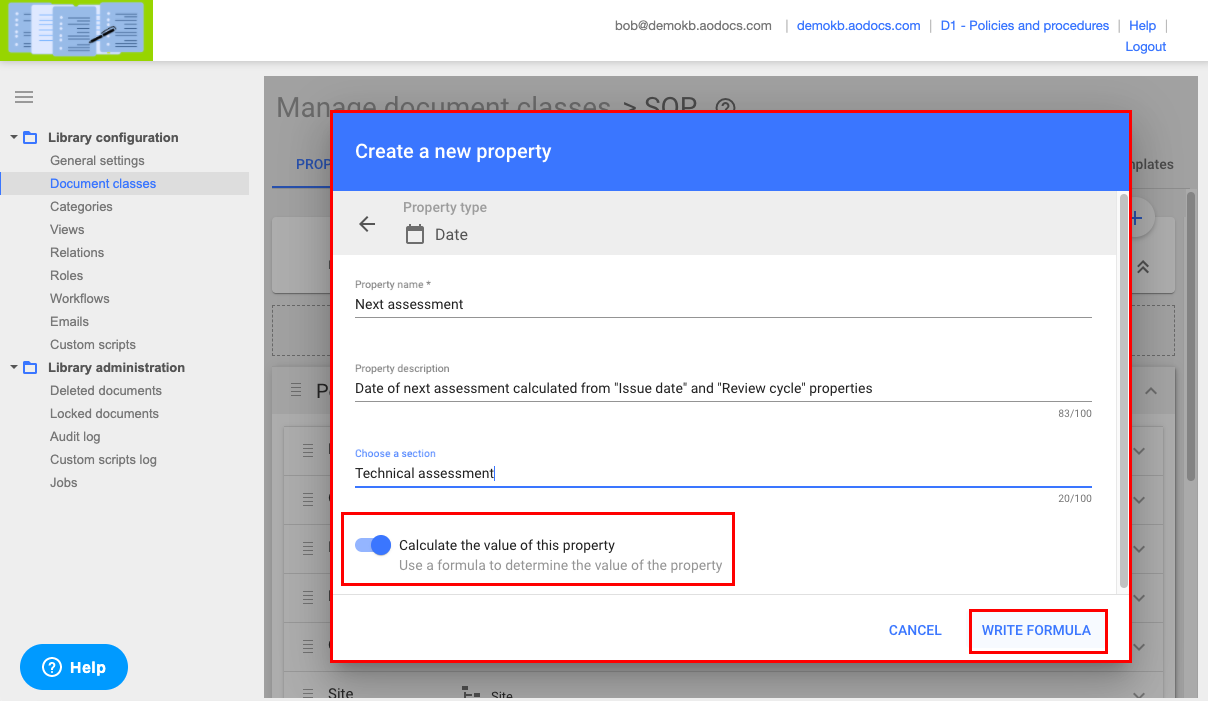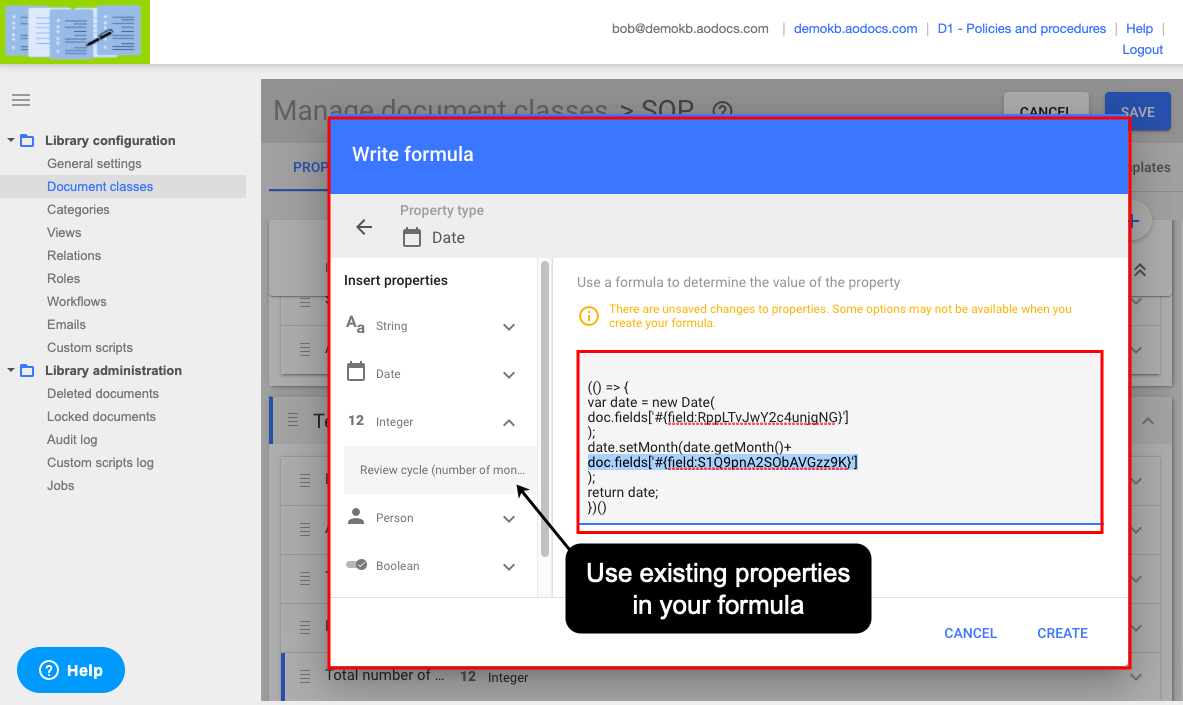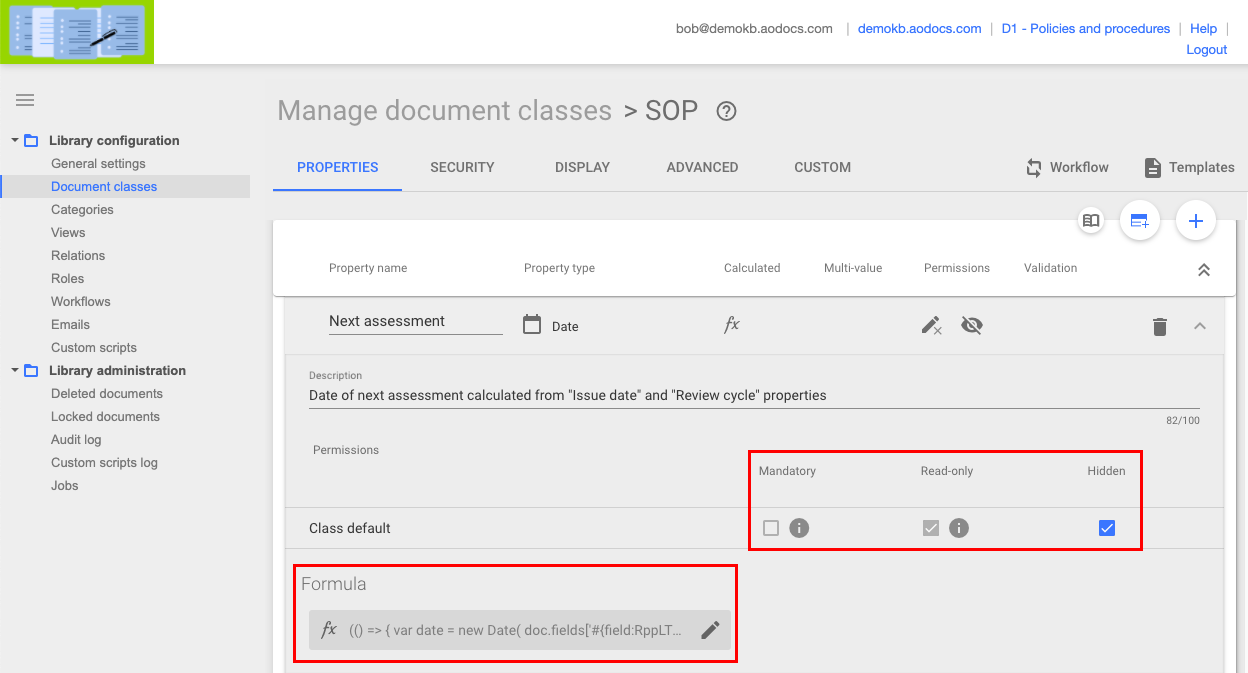# Create and configure calculated properties

Calculated properties are custom properties whose values are calculated from a formula. The formula can include the values of other properties, thereby creating dependencies between your properties.

Notes:
– This feature is currently available as a private beta and will be available as a public beta at a later date.

Examples of use cases:

• If you have a property named "Price of product", you can create a calculated property named "Value Added Tax" to calculate its VAT automatically when a user enters a value in the "Price of product" property.
• You can create a calculated property named "Publication date" which records the date when the document is transitioned to "Published" by workflow action.

Library administrators can create calculated properties, for all property types and in all types of library.

– Getting started with formulas for calculated properties: for beginnersCreate calculated propertiesEdit calculated properties

## Create calculated properties

Important: Don't combine calculated properties with custom scripts

2. Follow the procedure to create a new property. In the Create a new property pop-up, activate the switch Calculate the value of this property.

3. Press Write formula.4. In the Write formula pop-up, enter a formula in the field. Write the formula in JavaScript with a maximum of 500 characters.

Notes:
– You can use existing properties in your formula: a block of text with a unique identifier is inserted into your formula. To use properties in your formula, they must already be saved. If you have created one or more properties and haven't yet saved them, they're not available in the Write formula pop-up.
– Don't use calculated properties as input to other calculated properties. However, you can use a calculated property in its own formula: the previous value of the calculated property will be used to calculate a new value.
– Your formula must be valid:
– if the syntax of your formula is invalid, you can't save the formula
– if the calculation of the value produces an error, users won't be able to save or
create documents in this document class5. Press Create.

6. Press Save

## Edit calculated properties

1. In the document class settings in the library administration, expand a calculated property to display its full information.

Note: Calculated properties can't be mandatory and are always read-only. You can't modify these two fields. However calculated properties can be hidden.

2. Press the pencil button under Formula. The Edit formula pop-up opens.

3. Edit your formula and press OK

4. Press Save.Note: If you didn't define a property as calculated when you created it, you can't define it as calculated in the user interface once you have saved it. This can be done only by API.